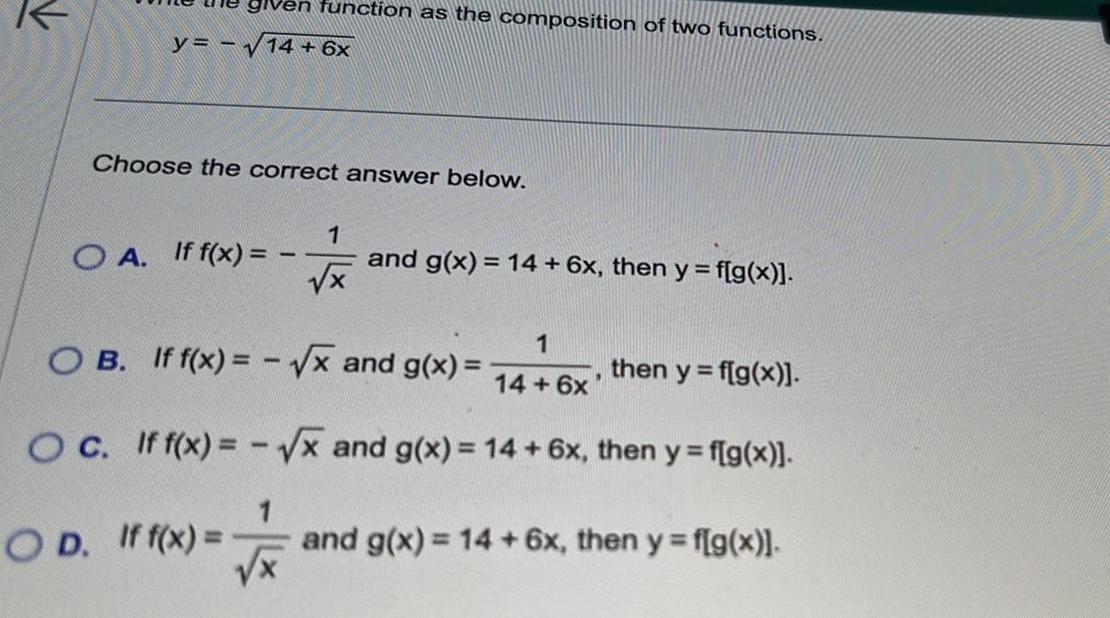Question:

# function as the composition of two functions y 14 6x Choose

Last updated: 9/8/2023function as the composition of two functions y 14 6x Choose the correct answer below OA If f x 1 1 x and g x 14 6x then y f g x 1 OB If f x x and g x then y f g x 14 6x OC If f x x and g x 14 6x then y f g x OD If f x and g x 14 6x then y f g x